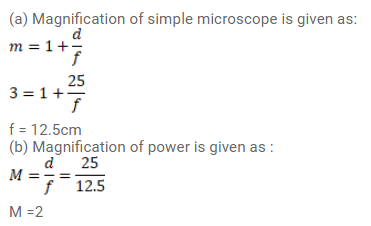# A simple microscope has a magnifying power of`
Question:

A simple microscope has a magnifying power of $3^{\prime} 0$ when the image is formed at the near point ( $\left.25 \mathrm{~cm}\right)$ of a normal eye.

(a) What is its focal length?

(b) What will be its magnifying power if the image is formed at infinity?

Solution: